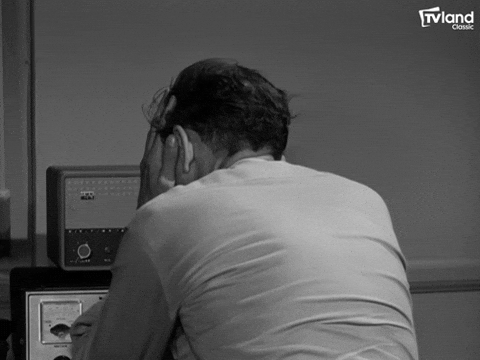## DEV Community 👩‍💻👨‍💻# Random Colors Generating random color with single line of js code

Did you ever feel bored or tired of writing long random colors for different divs or spans just to test something simple?So here is a simple solution. The following snippet generates a random color in hexadecimal format.

``````var randomColor = '#'+Math.floor(Math.random()*16777215).toString(16);
//generates a random color -> #56eec7
``````

That's it!🥳 You can place this in a function and call the function everytime you need a random color

``````function generateRandomColor()
{
var randomColor = '#'+Math.floor(Math.random()*16777215).toString(16);
return randomColor;
//random color will be freshly served
}
document.body.style.backgroundColor = generateRandomColor() // -> #e1ac94
someDiv.style.color = generateRandomColor() // -> #34c7aa
``````

Well this is the end of the trick. If you are in hurry, you can the leave post here.

# But,

if you are curious to know why only the number 16777215 and toString(16) are used, then the following part covers those explanations

So, let's divide the code into 3 parts

### 1.Why the number `16777215`?

• Well this needs a little bit of Math. We all know that the colors range from `#000000`(pitch black) to `#ffffff`(pure white).
• The number of colors that exist from black to white as per rgb values are `16777216`.
• This can be calculated simply by using permutation&combination formula `[result = m to the power of n => 16 to power of 6 => 16777216]`
• However our ultimate goal is to convert the number into hexadecimal format and `16777216` converts to `1000000` and `16777215` converts to `ffffff`. Hence we proceed with 167777215 as the highest number for hexadecimal conversion

### 2.Randomness

• As we need some randomness in our output we are multiplying our magic number with `Math.random()` which returns floating number in range from inclusive of 0 to exclusive of 1
``````Math.random()*16777215
//->9653486.355498075
``````
• As seen the output is floating point and we need to cut down it to an integer for hex conversion and hence we use `Math.floor()` for that
``````Math.floor(Math.random()*16777215)
//->96953486
``````

• Now we are in the endgame, the last part of the code. To convert a number to hexadecimal format string , we have a beautiful method `toString()` which accepts the number that tells to which format it has to convert.
• As we are converting to string of hexa-decimal format and hence we pass 16 as the argument as follows
``````(96953486).toString(16)
//->934cee
Math.floor(Math.random()*16777215).toString(16);
//->12ef556
``````
``````- All we need to now is just attach # before the string
``````
``````var randomColor = '#'+Math.floor(Math.random()*16777215).toString(16);
//->#19feac
``````

That's it!
Kudos to you!
You have successfully completed the post
Happy C0ding!Maria Campbell

I discovered this too, and it's great. However, occasionally it generates a hex code that contains only 5 characters instead of 6. But if you chain ES6's .padStart() method to the end of the code like so:

``````const randomColor = Math.floor(Math.random()*16777215).toString(16).padStart(6, '0')
``````

This ensures that a 6 character hex color code is always generated. Thanks for the explanation about the number 16777215. I was wondering about that part!

This is where I learned about using ES6's padStart() method to fix the hex color code length issue: stackoverflow.com/questions/148450...I think there's a bug so you'll only get the colors #000000 - #FFFFFE, never #FFFFFF. Because the max value Math.random can return is 0.999… and not 1.0, `Math.random()` multiplied with some integer n will always be less than n, and rounded down you'll get 0…n-1, not 0…n. For instance Math.floor(Math.random()) will always be 0 because `Math.floor(0.999…)` is still 0, and `Math.floor(Math.random() * 10)` will never be 10, because `Math.floor(9.999…)` is 9.

If you want every possible color you should probably use `Math.floor(Math.random()*16777216)` (or `Math.floor(Math.random()*0x1000000)` which I think is more readable).Andrej Lacko

why you write post buggy? it is not so hard to test it

try

``````'#'+Math.floor(0.00000001*16777215).toString(16);
``````

returns '#0'

try

``````'#'+Math.floor(0.9999999999999*16777215).toString(16);
``````

returns '#fffffe'
so you will never get '#ffffff'

this is solution I like the most, because you know, what it does

``````'#' + Math.floor(Math.random() * (256 * 256 * 256)).toString(16).padStart(6, '0')
``````

it is longer, but human readable and you can test it pretty easily, similar to as Maria Campbell

if you want to be nerdy looking, shorter solution, here it is

``````'#'+Math.random().toString(16).substr(2,6)
``````

but trust me, you will get headache, before you decipher it

## Visualizing Promises and Async/Await 🤯☝️ Check out this all-time classic DEV post